﻿ Variational Iteration Method for a Singular Perturbation Boundary Value Problems

### Variational Iteration Method for a Singular Perturbation Boundary Value Problems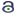OPEN ACCESS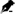PEER-REVIEWED

## Variational Iteration Method for a Singular Perturbation Boundary Value Problems

Hradyesh Kumar Mishra1,, Sonali saini1,1Department of Mathematics, Jaypee University of Engineering & Technology, Guna (M.P), India

### Abstract

In this paper, the author used He’s variational iteration method for solving singularly perturbed two-point boundary value problems. Few examples are solved to demonstrate the applicability of the method. It is observed that a good choice of the freely selected initial approximation in the variational iteration method leads to closed form solutions by using only one or two iterations. It is also observed that the variational iteration method can be easily applied to the initial and boundary value problems. Graphs are also plotted for the numerical examples.

### At a glance: Figures

1
Prev Next

• Mishra, Hradyesh Kumar, and Sonali saini. "Variational Iteration Method for a Singular Perturbation Boundary Value Problems." American Journal of Numerical Analysis 2.4 (2014): 102-106.
• Mishra, H. K. , & saini, S. (2014). Variational Iteration Method for a Singular Perturbation Boundary Value Problems. American Journal of Numerical Analysis, 2(4), 102-106.
• Mishra, Hradyesh Kumar, and Sonali saini. "Variational Iteration Method for a Singular Perturbation Boundary Value Problems." American Journal of Numerical Analysis 2, no. 4 (2014): 102-106.

 Import into BibTeX Import into EndNote Import into RefMan Import into RefWorks

### 1. Introduction

We consider the following class of singularly perturbed two-point boundary value problems (BVPs)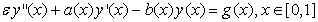(1)

Boundary conditions are(2)

Whereis a small positive parameter (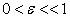),are given constants. andare assumed to be sufficiently continuously differentiable function in [0,1] and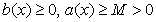on [0,1] where M is some positive constant. Under these assumptions (1)-(2) has a unique solution. This type of boundary value problems are generally occurs in optimal control theory, reaction-diffusion process, fluid mechanics and quantum mechanics. There are so many technique for solving singular perturbation problems [5, 22, 24, 26].

The variational iteration method (VIM) was proposed by Ji-Huan He [7-14]. The idea of VIM is to construct a correction functional using generalized Lagrange multipliers. The multipliers can be defined using the calculus of variations. The multipliers in the correction functional should be chosen such that the corrected solution is superior to the initial approximation (trial function) and is the best for the given type of trial function. The trial function can be freely chosen with possible unknowns, which can be determined by imposing the boundary/initial conditions. The method has been found to give rapidly convergent successive approximations for the solution if such a solution exists. The VIM is used by many researchers to study different type of equation for finding both analytical and approximate solution. VIM method is used for solving autonomous ordinary differential system in He, Fisher’s equation, Klein-Gorden equation, Voletra and Fredholm Equation and many linear and non-linear equations. Some author used the variational iteration method in many other problems, for example Dehghan and Shakeri  applied VIM to solve the Cauchy reaction-diffusion problem. Jinbo and Jiang  used VIM to solve an inverse parabolic equation. H.K.Mishra  studied a comparative study of variational iteration method and He Laplace method. Ramos  applied VIM to solve nonlinear partial differential equations. Das  used VIM to obtain the solution of a fractional-diffusion equation. Assas  applied VIM to solve coupled-KdV equations. Mustafa etc  used VIM to solve space and time-fractional Burgers equations with initial conditions. Javidi and Jalilian  applied VIM to calculate the wave solution of the Boussinesq equation. Wazwaz  applied VIM to some linear and nonlinear systems of PDEs. Batiha et al.  used VIM to solve systems of PDEs. Salkuyeh  showed convergence of VIM for linear systems of ODEs with constant coefficients.

In this paper, the goal of our study is to introduce He’s Variational Itreation Method to solve a singular singularly perturbed two-point boundary value problems (BVPs). In the section 2, analysis of variation iteration method is given. Section 3 of the paper is based on some numerical examples for showing the utility of the presented method. In the last section 4, we will discuss our results with conclusion.

### 2. Analysis of Variational Iteration Method

By He’s method we introduce the following correction functional corresponding to equation (1)(3)

Whereis a Lagranges multiplier that can be identify by VIM method. After using the restricted variationswe obtain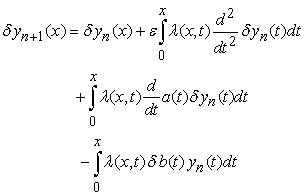(4)

Integrating (4) by parts, we get(5)

After solving the equation with imposing the above restricted variation terms to (5) we obtain the following Euler- Langrange equation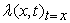(6)

### 3. Numerical Results

To incorporate our discussion above two special cases of the singularly perturbed two-point boundary value problem (1)-(2) will be studied. Here we made comparison between one iteration Variational iteration method and the exact solution for each problem. All the results are calculated by using Mathematica software.

Example 3.1. Consider the following singular perturbation problem from Bender and Orszag [3, p. 480, problem 9.17 with]:(7)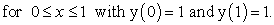(8)

The exact solution is given by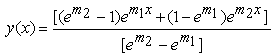(9)

where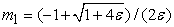and.

Solving (6) using the coefficients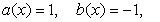thencan easily identified as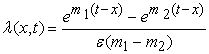HereTherefore, we have the following iteration formula,Now, we begin with the following initial approximation,where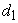and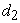are constants to be determined.

By the above iteration formula, we haveApplying the boundary conditions,we have atatHence#### Table 1.

The numerical results of examples 3.1 are given in the Table 1 for.

The numerical results of examples 3.1 are given in the Table 2 for#### Table 2.

Figure 1. Error analysis for Table 1 atExample 3.2. Consider the following homogeneous SPP from Kevorkian and Cole [6, p.33, Eqs. (2.3.26) and (2.3.27) with],(10)(11)

The exact solution is given by(12)

Solving (6) using the coefficientsthencan easily identified aswhereandare constants to be determined.

By the above iteration formula, we have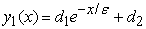Applying the boundary conditions yield,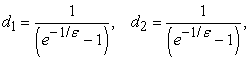Thus,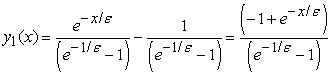which is the exact solution.

### 4. Conclusion

We have described a variational iteration method for solving singularly perturbed boundary value problem. In Table 1 and Table 2 we have shown the maximum error for the problems using variational iteration method. As we decrease the value of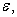to study the behavior of the solution at the boundary layer we need a large number of mesh points in that region. Therefore the uniform mesh is not a good technique for such problems because the number of mesh points in the boundary layer region should be much higher than that from the outer region. Figure 1-Figure 2 has been plotted for values ofbelong toversus the computed solution obtained at the different value of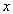for different value of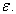### References

  Abdul-Majid Wazwaz, The variational iteration method for solving linear and nonlinear systems of PDEs, Computers and Mathematics with Applications, 54 (2007), 895-902.In article CrossRef  B.Batiha,M.S.M. Noorani, I. Hashim, K. Batiha, Numerical simulations of systems of PDEs by variational iteration method, Physics Letters A, 372, (2008), 822-829.In article CrossRef  C. M. Bender and S. A. Orszag, “Advanced Mathematical Methods for Scientists and Engineers,” McGraw-Hill, New York, 1978.In article  Davod Khojasteh Salkuyeh, Convergence of the variational iteration method for solving linear systems of ODEs with constant coefficients, Computers and Mathematics with Applications, 56 (2008), 2027-2033.In article CrossRef  J. Jayakumar, Improvement of numerical solution by boundary value technique for singularly perturbed one-dimensional reaction diffusion problem, Applied Mathematics and Computation, 142 (2003), 417-447.In article CrossRef  J. Kevorkian, J.D. Cole, Perturbation Methods in Applied Mathematics, Springer-Verlag, New York (1981).In article CrossRef  J. He, A New Approach to Nonlinear Partial Differential Equations, Communication Nonlinear Science & Numerical Simulation, Vo1.2, No.4 (Dec. 1997), 230-235.In article  J.H. He, Approximate analytical solution for seepage flow with fractional derivatives in porous media, Comput. Methods Appl. Mech. Eng. 167 (1998) 57-68.In article CrossRef  J.H. He, Approximate solution of nonlinear differential equations with convolution product nonlinearities, Comput. Methods Appl. Mech. Eng. 167 (1998) 69-73.In article CrossRef  J.H. He, Variational iteration method-a kind of non-linear analytical technique: some examples, Internat. J. Nonlinear Mech. 34 (1999) 699-708.In article CrossRef  J.H. He, Variational iteration method for autonomous ordinary differential systems, Appl. Math. Comput. 114 (2000) 115-123.In article CrossRef  J. He, Homotopy perturbation method: a new nonlinear analytical technique, Applied Mathematics and Computation 135 (2003) 73-79.In article CrossRef  J.H. He, The homotopy perturbation method for nonlinear oscillators with discontinuities, Appl. Math. Comput. 151 (2004) 287-292.In article CrossRef  J.H. He, Variational iteration method—Some recent results and new interpretations, J. Comput. Appl. Math. in press.In article  J.I. Ramos, On the variational iteration method and other iterative techniques for nonlinear differential equations, Applied Mathematics and Computation, 199 (2008), 39-69.In article CrossRef  Laila M.B. Assas, Variational iteration method for solving coupled-KdV equations, Chaos, Solitons and Fractals, 38 (2008), 1225-1228.In article CrossRef  Liu Jinbo, Tang Jiang, Variational iteration method for solving an inverse parabolic equation, Physics Letters A., 372 (2008), 3569-3572.In article CrossRef  M. Javidi, Y. Jalilian, Exact solitary wave solution of Boussinesq equation by VIM, Chaos, Solitons and Fractals, 36 (2008), 1256-1260.In article CrossRef  Mehdi Dehghan, Fatemeh Shakeri, Application of He’s variational iteration method for solving the Cauchy reaction- diffusion problem, Journal of Computational and Applied Mathematics., 214 (2008), 435-446.In article CrossRef  H.K.Mishra, A comparative study of variational iteration method and He Laplace method, Applied Mathematics, 2012, 3, pp. 1193-1201.In article CrossRef  H.K. Mishra., Initial value technique for singular perturbation boundary value problems, American Journal of Numerical Analysis, 2 (2014), 49-54.In article  Hradyesh Kumar Mishra, Sonali Saini, Numerical Solution of Singularly Perturbed Two-Point Boundary Value Problem via Liouville-Green Transform, American Journal of Computational Mathematics, 3(2013)1-5.In article CrossRef  Mustafa Inc, The approximate and exact solutions of the space- and time fractional Burgers equations with initial conditions by variational iteration method, J. Math. Anal. Appl., 345 (2008), 476-484.In article CrossRef  R.E. O’Malley, Introduction to Singular Perturbations, Academic Press, New York (1974).In article  S. Das, Analytical solution of a fractional diffusion equation by variational iteration method, Computers and Mathematics with Applications, 57 (2009), 483-487.In article CrossRef  Y.N. Reddy, P. Pramod Chakravarthy, An initial-value approach for solving singularly perturbed two-point boundary value problems, Applied Mathematics and Computation, 155, No. 1 (2004), 95-110.In article CrossRef